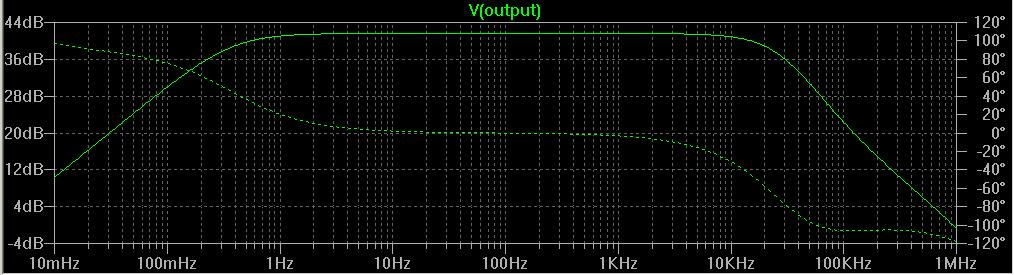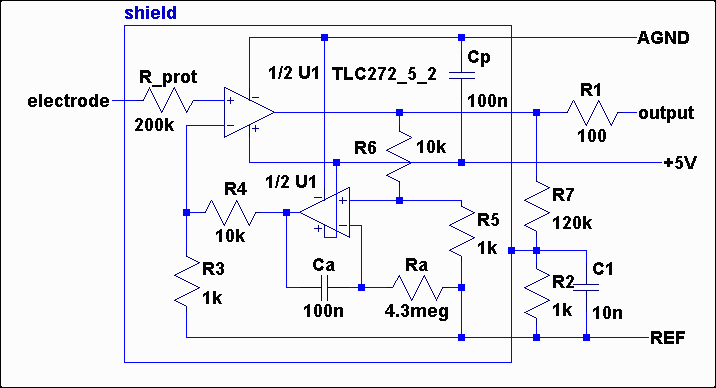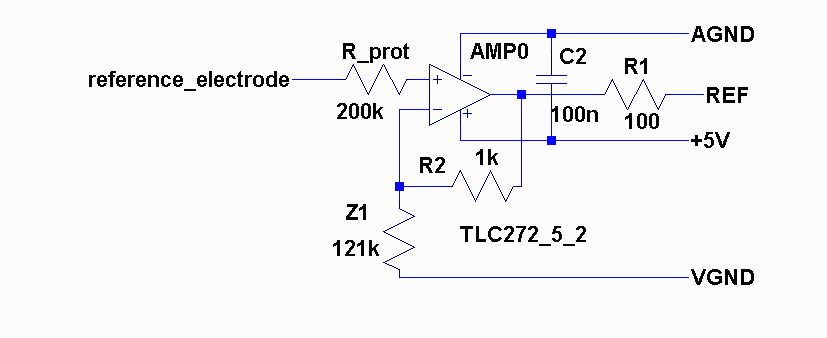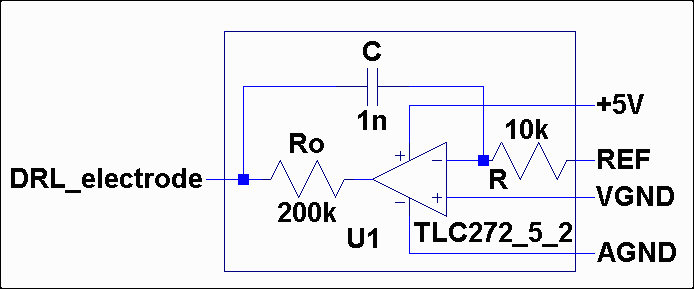Although the prototype 2 has good results, I started to think on next design (*).

Because:
• Previous design doesn't amplify the signal, therefore the connection from electrodes to modularEEG is still sensitive to intereferences. Especially if the cables are longer.
• It should be relatively easy to add gain with using new design below, and the number of parts (comparing to prototype 2) would increase not that much (just 5-7 resistors more). Considering the fact, that it already has HF rejection, it is even less.

• If this new design works, then modularEEG could be simplified greatly. That would mean, that it could either hold more channels on one board, or could be miniaturized and become more portable.
• With simplification of modularEEG (INA114 and input ESD protection taken out), the total cost would drop significantly (INA114 is expensive).
• For each new channel, only one active electrode is needed, because the reference active electrode is common. Therefore for more then one channel it is cheaper and more effective (less electrodes on head required) then in prototype 2.

Points to consider:
• It will probably not work with current modularEEG. Perhaps it could work if INA114 was taken out, gain decreased and active electrode output connected at "Electrode DC checkpoint" (see modularEEG analog board scheme). If the INA114 is in socket, this could be done without any soldering. DRL from modularEEG could be used too, it is identical as in the design here.
• If new (simplified) version of modularEEG was built, it would not work with passive electrodes
• It needs to be built and verified.

 LTSpice simulationAmplifier electrodeReference electrodeDRL electrodeCommon mode gain should be zero. To achieve that, this condition must be met:
Z1 * R3 * R5 = R2 * (R4*R5 + R3*R6 + R4*R6)

Then the differential gain in the passband is:
G = (R4/R3 + 1) * (R6/R5 + 1)

CMRR = 20 log (50*G/tol)
where tol is resistor tolerance in %

For example with gain G=1000 and tolerance 1%, the CMRR is 94dB (DRL will add to that about 40dB).

For the elements values in the above schemes, the differential gain G is 121. It needs yet to be decided what would be the best value. To achieve higher CMRR, resistors 0.1% could used, but they are expensive.

*The design is based on article "Instrumentaion Amplifiers for bioelectric events: a design with a minimal number of parts" A.C.MettingVanRijn, A.Peper, C.A.Grimbergen